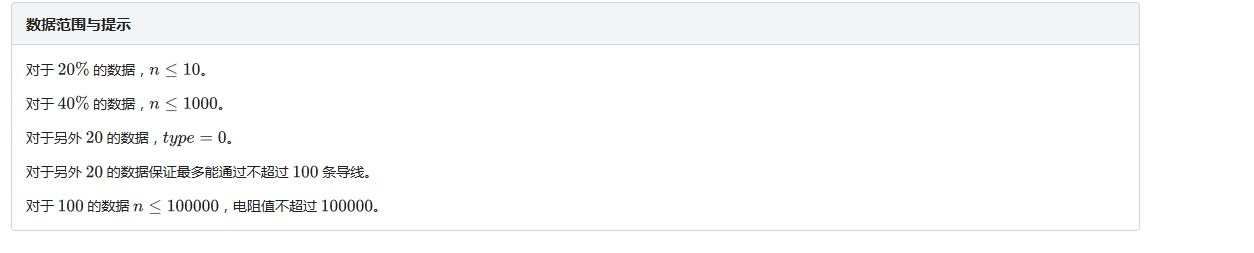﻿ 2020 5 27 树状数组丶Java教程网-IT开发者们的技术天堂• 111541

文章

• 803

评论

• 12

友链

## 2020 5 27 树状数组这是一个比较显然的dp，最长不下降子序列。

CODE

``````#include<bits/stdc++.h>
#define ll long long
using namespace std;
const int mod=123456789;
{
char c=getchar();ll a=0,b=1;
for(;c<'0'||c>'9';c=getchar())if(c=='-')b=-1;
for(;c>='0'&&c<='9';c=getchar())a=a*10+c-48;
return a*b;
}
int n,type,a;
struct tree
{
int v,s;//长度，方案数
inline void clear()
{
v=0;
s=1;
return;
}
}t;
int lowbit(int x)
{
return x&(-x);
}
inline int change(int x,tree y)
{
while(x<=100000)
{
if(t[x].v<y.v)
{
t[x]=y;
}
else
{
if(y.v==t[x].v)
{
t[x].s+=y.s;
t[x].s%=mod;
}
}
x+=lowbit(x);
}
}
{
tree res;
res.clear();
while(x>0)
{
if(t[x].v>res.v)
{
res=t[x];
}
else
if(res.v==t[x].v)
{
res.s+=t[x].s;
res.s%=mod;
}
x-=lowbit(x);
}
return res;
}
inline tree changeans(tree x,tree y)
{
if(x.v<y.v)
{
return y;
}
else
{
if(x.v==y.v)
{
x.s+=y.s;
x.s%=mod;
return x;
}
}
return x;
}
int main()
{
tree ans;ans.clear();
for(int i=1;i<=n;i++)
{
x.v++;
ans=changeans(ans,x);
change(a[i],x);
}
cout<<ans.v%mod<<endl;
if(type==1)cout<<ans.s%mod<<endl;
return 0;
}``````

| 喜欢本站的朋友可以收藏本站,或者加入我们大家一起来交流技术!『数据结构总结1：树状数组』

## ZhaoWei-2020-01-27

ZhaoWei-2020-01-27

## 【Java - J - 27】二叉树的镜像

【Java - J - 27】二叉树的镜像

## 初学Nuxt.js之Vuex状态树

### 0条评论

•闹市重声(默认)
•守望先锋
•水滴玻璃
•黄金分割
•黄昏花丛
•我的后宫
•死萝莉控
•末花未名